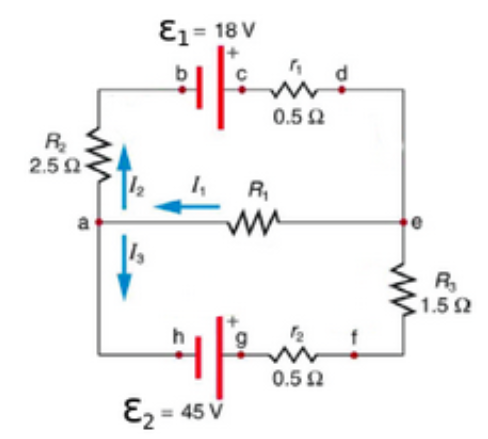# Problem: Consider the circuit diagram depicted in the figurePart (a) What equation do you get when you apply the loop rule to the loop abcdefgha?Part (b) If the current through the top branch is I2 = 0.49 A, what is the current through the bottom I3, in amps?

###### FREE Expert Solution

Loop rule:

$\overline{){\mathbf{\sum }}{\mathbf{V}}{\mathbf{=}}{\mathbf{0}}}$

91% (345 ratings)###### Problem Details

Consider the circuit diagram depicted in the figurePart (a) What equation do you get when you apply the loop rule to the loop abcdefgha?

Part (b) If the current through the top branch is I2 = 0.49 A, what is the current through the bottom I3, in amps?